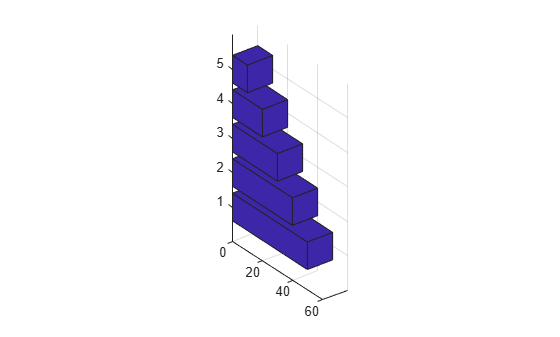# bar3h

Plot horizontal 3-D bar graph## Syntax

```bar3h(Y) bar3h(Z,Y) bar3h(...,width) bar3h(...,style) bar3h(...,color) bar3h(ax,...) h = bar3h(...) ```

## Description

`bar3h` draws three-dimensional horizontal bar charts.

`bar3h(Y)` draws a three-dimensional bar chart, where each element in `Y` corresponds to one bar. When `Y` is a vector, the z-axis scale ranges from `1` to `length(Y)`. When `Y` is a matrix, the z-axis scale ranges from `1` to the number of rows in `Y`.

`bar3h(Z,Y)` draws a bar chart of the elements in `Y` at the locations specified in `Z`, where `Z` is a vector defining the z values for the horizontal bars. The z values can be nonmonotonic, but cannot contain duplicate values. If `Y` is a matrix, elements from the same row in `Y` appear at the same location along the `z`-axis.

`bar3h(...,width)` sets the width of the bars and controls the separation of bars within a group. The default `width` is `0.8` and the bars have a slight separation. If `width` is `1`, then the bars within a group touch one another.

`bar3h(...,style)` specifies the style of the bars. `style` is `'detached'`, `'grouped'`, or `'stacked'`. Default mode of display is `'detached'`.

• `'detached'` displays the elements of each row in `Y` as separate blocks behind one another in the x direction.

• `'grouped'` displays n groups of m horizontal bars, where n is the number of rows and m is the number of columns in `Y`. Each group contains one bar per column in `Y`.

• `'stacked'` displays one bar for each row in `Y`. The bar length is the sum of the elements in the row. Each bar is multicolored, with colors corresponding to distinct elements and showing the relative contribution each row element makes to the total sum.

`bar3h(...,color)` displays all bars using the color specified by `color`. For example, use `'r'` for red bars. Specify `color` as one of these values: `'r'`, `'g'`, `'b'`, `'c'`, `'m'`, `'y'`, `'k'`, or `'w'`.

`bar3h(ax,...)` plots into the axes `ax` instead of into the current axes (`gca`).

`h = bar3h(...)` returns a vector `Surface` objects. When `Y` is a matrix, `bar3h` creates one `Surface` object per column in `Y`.

## Examples

collapse all

Load the data set `count.dat`, which returns a three-column matrix, `count`. Store `Y` as the first ten rows of `count`.

```load count.dat Y = count(1:10,:);```

Create a 3-D horizontal bar graph of `Y`. By default, the style is `detached`.

```figure bar3h(Y)```Load the data set `count.dat`, which returns a three-column matrix, `count`. Store `Y` as the first ten rows of `count`.

```load count.dat; Y = count(1:10,:);```

Create a 3-D horizontal bar graph of `Y` and set the bar width to 0.5.

```width = 0.5; figure bar3h(Y,width) title('Width of 0.5')```Load the data set `count.dat`, which returns a three-column matrix, `count`. Store `Y` as the first ten rows of `count`.

```load count.dat Y = count(1:10,:);```

Create a 3-D horizontal bar graph of Y and specify the style option as `grouped`.

```figure bar3h(Y,'grouped') title('Grouped Style Option')```Load the data set `count.dat`, which returns a three-column matrix, `count`. Store `Y` as the first ten rows of `count`.

```load count.dat Y = count(1:10,:);```

Create a 3-D horizontal bar graph of Y and specify the style option as `stacked`.

```figure bar3h(Y,'stacked') title('Stacked Style Option')```